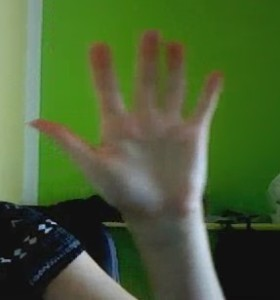Posted on

# Visual Finger Counter

Gabriela Brndiarova

Aim of this project was implementing of finger counter with OpenCV. Input from ordinary webcam was used and it is possible to get realtime results, now. At first, we segmented hand using camshift algorithm. After that, we got hand contours and convexity defect. There was used very simple algorithm to count fingers when the convexity defects were known.

Function used: calcHist, calcBackProject, CamShift, threshold, morphologyEx, findContours, convexHull, convexityDefects

## Input## The process

1. Selecting a litle square on the hand with the mouse.
2. Calculating histogram from selected range.
```Mat frame, hsv, hue, mask, hist = Mat::zeros(200, 320, CV_8UC3);
inRange(hsv, Scalar(0, smin, 10), Scalar(180, 256, 256), mask);
hue.create(hsv.size(), hsv.depth());
mixChannels(&hsv, 1, &hue, 1, ch, 1);
calcHist(&roi, 1, 0, maskroi, hist, 1, &hsize, &phranges);
```
3. Getting back projection of image.
```calcBackProject(&hue, 1, 0, hist, backproj, &phranges);
```
4. Camshift application to get selection of hand.
```CamShift(backproj, trackWindow, TermCriteria( CV_TERMCRIT_EPS | V_TERMCRIT_ITER, 10, 1 ));
```
5. Manual making the selection bigger (it is important to have whole fingers in the selection), but not too big because of face. If face is in the selection, hand segmentation is no longer possible.
6. The selection of hand is cut off and rotate to natural position for human (fingers point to the top).
```int angle = trackBox.angle;
Size rect_size = trackBox.size;
if (angle >90){
angle -= 180;
angle *= -1;
}
M = getRotationMatrix2D(trackBox.center, angle, 1.0);
```
7. Treshold application.
```threshold(croppedMask, croppedMask, tresholdValue, 255 , THRESH_BINARY);
```
8. Morphology closing application.
```Mat structElem = getStructuringElement(MORPH_ELLIPSE, Size(elemSize,elemSize));
```
9. Getting all contours and selecting the longest of them â€“ contour of hand. Short contours are just contours of some kind of noise.
```vector<vector<Point> > contours;
vector<Vec4i> hierarchy;

double largestArea = 0.0;
int largestContourIndex = 0;
for( int i = 0; i< contours.size(); i++ ){
double a=contourArea( contours[i],false);
if(a>largestArea){
largestArea=a;
largestContourIndex=i;
}
}
```
10. Getting convexity defects.
```vector<vector<int> > hulls (1);
convexHull(contours[largestContourIndex], hulls, false, false);
std::vector<Vec4i> defects;
convexityDefects(contours[largestContourIndex], hulls, defects);
```
11. Counting fingers using convexity defects. We do not count too small convexity defects, defects with too long distance between start and end point and too small distance, too. This is the way how to filter defects between fingers. Number of finger is always number of defects plus 1. It is not the best way but for purposes of this project it is good.
```int fingerCount = 1;
for (int i = 0; i< defects.size(); i++){
int start_index = defects[i];
CvPoint start_point = contours[largestContourIndex][start_index];
int end_index = defects[i];
CvPoint end_point = contours[largestContourIndex][end_index];
double d1 = (end_point.x - start_point.x);
double d2 = (end_point.y - start_point.y);
double distance = sqrt((d1*d1)+(d2*d2));
int depth_index = defects[i];
int depth =  defects[i]/1000;

if (depth > 10 && distance > 2.0 && distance < 200.0){
fingerCount ++;
}
}
```
12. Previous steps are running really fast so it is not possible to show new result after every single iteration. The result can be change because of small mistake or noise. This is reason why we decided to show average value of last 15 cycles as result.
```countValue[iCV%15] = itCount;
iCV++;

int count = 0;
for (int i=0; i<15; i++){
count += countValue[i];
}
count = count/15;

stringstream ss;
ss << count;
string str = ss.str();
Point textOrg(10, 130);
putText(input, str, textOrg, 1, 3, Scalar(0,255,255), 3);
```